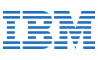New update is available. Click here to update.
Topics

# Jump Game

Moderate0/80
Average time to solve is 25m+8 more companies

## Problem statement

There is an array 'JUMP' of size 'N' which is 1-indexed and you are currently at index 1. Your goal is to reach index 'N' (end).

When you are at index 'i', you can jump a maximum length of 'JUMP[i]' which means you can make a jump of size 1 to JUMP[i]. Return true if you can reach the end otherwise false.

Example:-
``````N = 5
JUMP = [1,2,3,4,5]

ANSWER:- The answer should be YES as you can jump from 1st index to 2nd index, from 2nd index to 4th index, and from 4th index to 5th index.
``````
Detailed explanation ( Input/output format, Notes, Images )
Sample Input 1 :
``````4
4 4 4 4
``````
Sample Output 1 :
``````YES
``````
Explanation for Sample Output 1 :
``````It is YES because you can directly jump from the 1st position to the 4th position.
``````
Sample Input 2 :
``````3
1 0 2
``````
Sample Output 2 :
``````NO
``````
Explanation for Sample Output 2 :
``````It is NO because it is impossible to reach the 3rd position.
``````
Constraints :
``````1 <= N <= 10^5
1 <= JUMP[i] <= N

Time Limit = 1 sec
``````Console